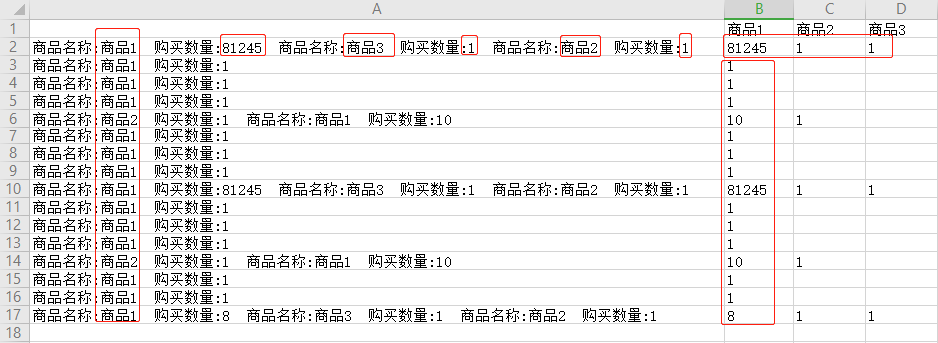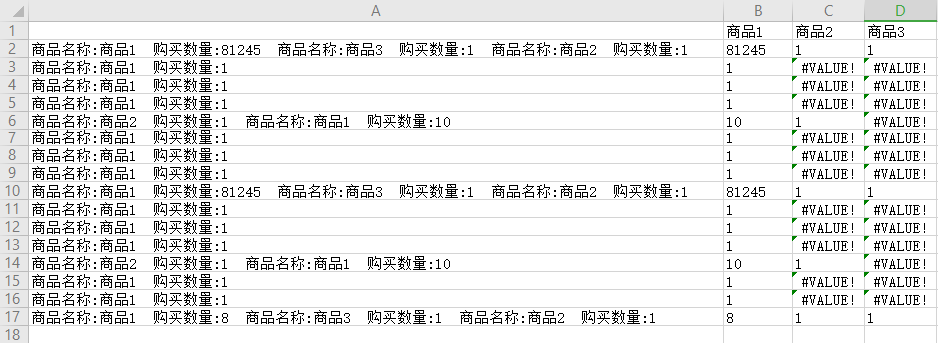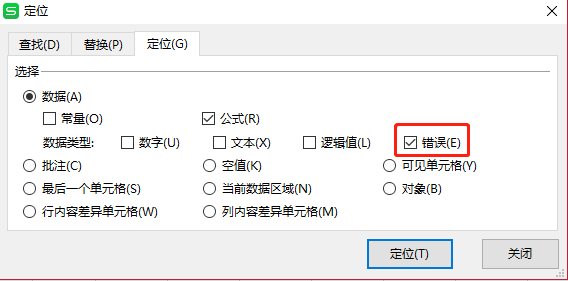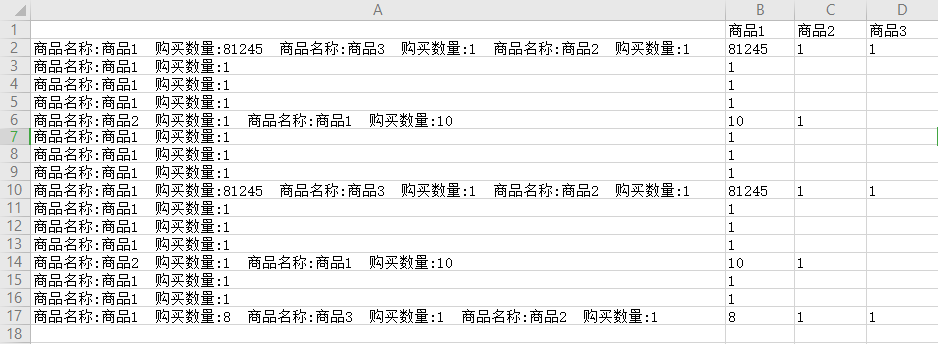# Excel:提取1.查找商品的下标：用FIND()函数

2.根据第1步的下标截取下标之后的字符串：用RIGHT()函数，计算字符串长度的时候用到了LEN()函数

3.计算第2步拿到字符串中空格的数量，分为等于0（A3数据）和大于0（A1数据）的情况，思路：

(LEN(B27)-LEN(SUBSTITUTE(B27," ","")))/LEN(" ")

4.判断第3步的空格数量，如果等于0结果则为第2步结果，如果大于0则先拿到第一个空格下标

截取字符串（第2步拿到的结果）0到第一个空格下标得到最终结果

1.FIND(B1,A2)，得到的结果是 6

2.RIGHT(A2,LEN(A2)-FIND(B$1,A2)-9)，得到的结果是 81245 商品名称:商品3 购买数量:1 商品名称:商品2 购买数量:1 3.(LEN(B27)-LEN(SUBSTITUTE(B27," ","")))/LEN(" ")，得到的结果是 8 4.找空格下标：FIND(" ",B27)（B27是上步所在的单元格），得到的结果是 6 根据第3步结果判断：IF(B28>0,LEFT(B27,FIND(" ",B27)),B27)，得到的结果是 81245 当然在过程中这样一步一步写太麻烦了，所以我们替换一下函数，得到最终函数 IF((LEN(RIGHT($A2,LEN($A2)-FIND(B$1,$A2)-9))-LEN(SUBSTITUTE(RIGHT($A2,LEN($A2)-FIND(B$1,$A2)-9)," ","")))/LEN(" ")>0,LEFT(RIGHT($A2,LEN($A2)-FIND(B$1,$A2)-9),FIND(" ",RIGHT($A2,LEN($A2)-FIND(B$1,$A2)-9))),RIGHT($A2,LEN($A2)-FIND(B$1,\$A2)-9))02-15150712-281万+
08-274344
02-016127
08-013198
07-181070
11-301万+
07-032645
04-23666
12-211123
11-077313
12-211308
07-22676
12-304091
03-293700
05-07609
02-17402
01-146161
12-042735